# 10.2 Non-right triangles: law of cosines  (Page 5/8)

 Page 5 / 8

Explain the relationship between the Pythagorean Theorem and the Law of Cosines.

When must you use the Law of Cosines instead of the Pythagorean Theorem?

The Law of Cosines must be used for any oblique (non-right) triangle.

## Algebraic

For the following exercises, assume $\text{\hspace{0.17em}}\alpha \text{\hspace{0.17em}}$ is opposite side $\text{\hspace{0.17em}}a,\beta \text{\hspace{0.17em}}$ is opposite side $\text{\hspace{0.17em}}b,\text{\hspace{0.17em}}$ and $\text{\hspace{0.17em}}\gamma \text{\hspace{0.17em}}$ is opposite side $\text{\hspace{0.17em}}c.\text{\hspace{0.17em}}$ If possible, solve each triangle for the unknown side. Round to the nearest tenth.

$\gamma =41.2°,a=2.49,b=3.13$

$\alpha =120°,b=6,c=7$

11.3

$\beta =58.7°,a=10.6,c=15.7$

$\gamma =115°,a=18,b=23$

34.7

$\alpha =119°,a=26,b=14$

$\gamma =113°,b=10,c=32$

26.7

$\beta =67°,a=49,b=38$

$\alpha =43.1°,a=184.2,b=242.8$

257.4

$\alpha =36.6°,a=186.2,b=242.2$

$\beta =50°,a=105,b=45{}_{}{}^{}$

not possible

For the following exercises, use the Law of Cosines to solve for the missing angle of the oblique triangle. Round to the nearest tenth.

$\text{\hspace{0.17em}}a=42,b=19,c=30;\text{\hspace{0.17em}}$ find angle $\text{\hspace{0.17em}}A.$

find angle $\text{\hspace{0.17em}}C.$

95.5°

$\text{\hspace{0.17em}}a=16,b=31,c=20;\text{\hspace{0.17em}}$ find angle $\text{\hspace{0.17em}}B.$

$\text{\hspace{0.17em}}a=13,\text{\hspace{0.17em}}b=22,\text{\hspace{0.17em}}c=28;\text{\hspace{0.17em}}$ find angle $\text{\hspace{0.17em}}A.$

26.9°

$a=108,\text{\hspace{0.17em}}b=132,\text{\hspace{0.17em}}c=160;\text{\hspace{0.17em}}$ find angle $\text{\hspace{0.17em}}C.\text{\hspace{0.17em}}$

For the following exercises, solve the triangle. Round to the nearest tenth.

$A=35°,b=8,c=11$

$B\approx 45.9°,C\approx 99.1°,a\approx 6.4$

$B=88°,a=4.4,c=5.2$

$C=121°,a=21,b=37$

$A\approx 20.6°,B\approx 38.4°,c\approx 51.1$

$a=13,b=11,c=15$

$a=3.1,b=3.5,c=5$

$A\approx 37.8°,B\approx 43.8,C\approx 98.4°$

$a=51,b=25,c=29$

For the following exercises, use Heron’s formula to find the area of the triangle. Round to the nearest hundredth.

Find the area of a triangle with sides of length 18 in, 21 in, and 32 in. Round to the nearest tenth.

177.56 in 2

Find the area of a triangle with sides of length 20 cm, 26 cm, and 37 cm. Round to the nearest tenth.

$a=\frac{1}{2}\text{\hspace{0.17em}}\text{m},b=\frac{1}{3}\text{\hspace{0.17em}}\text{m},c=\frac{1}{4}\text{\hspace{0.17em}}\text{m}$

0.04 m 2

0.91 yd 2

## Graphical

For the following exercises, find the length of side $x.$ Round to the nearest tenth.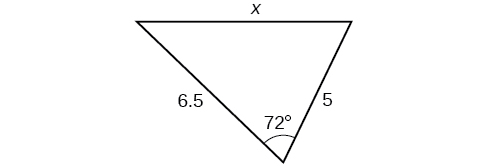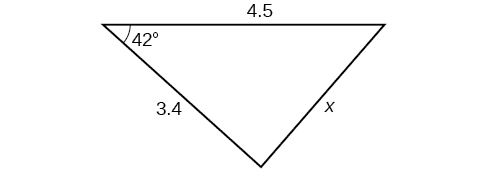3.0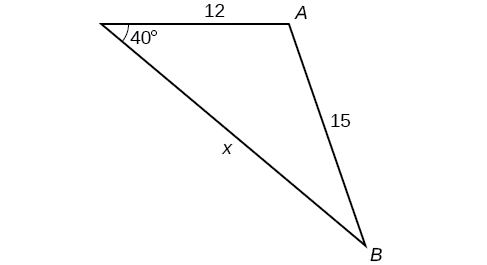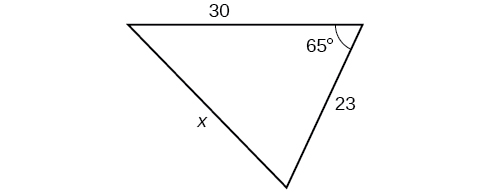29.1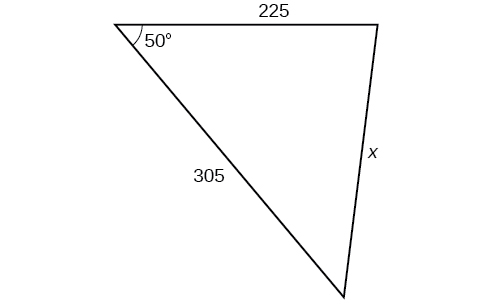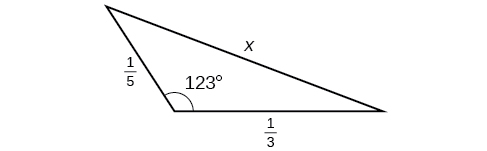0.5

For the following exercises, find the measurement of angle $\text{\hspace{0.17em}}A.$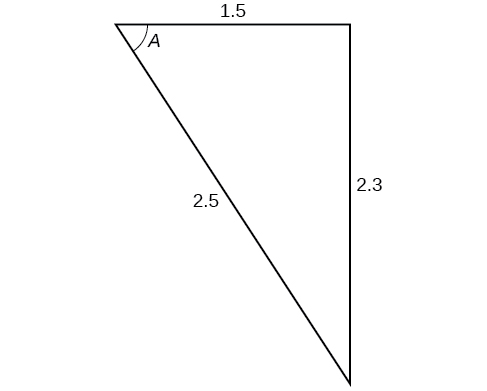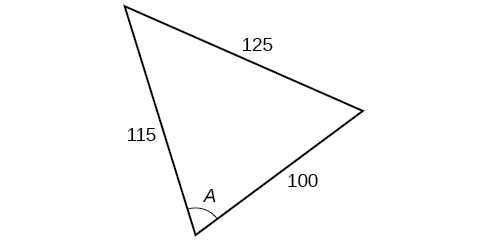70.7°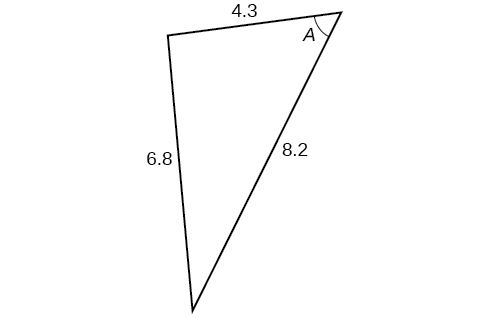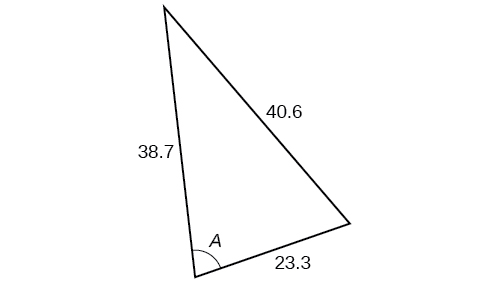77.4°

Find the measure of each angle in the triangle shown in [link] . Round to the nearest tenth.

For the following exercises, solve for the unknown side. Round to the nearest tenth.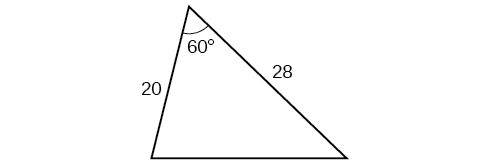25.0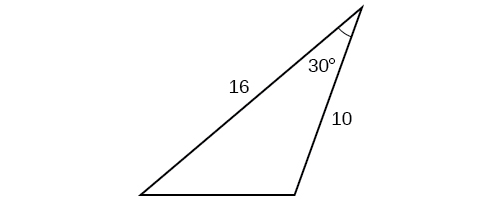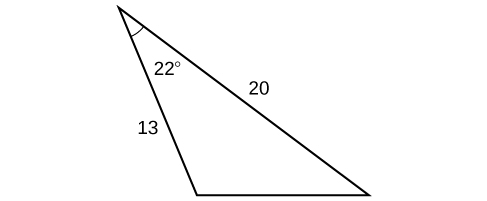9.3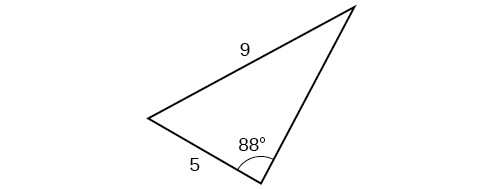For the following exercises, find the area of the triangle. Round to the nearest hundredth.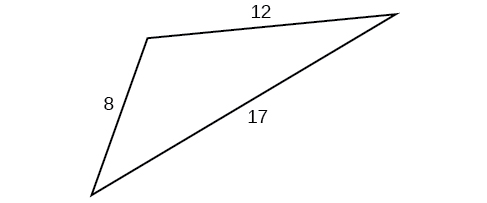43.52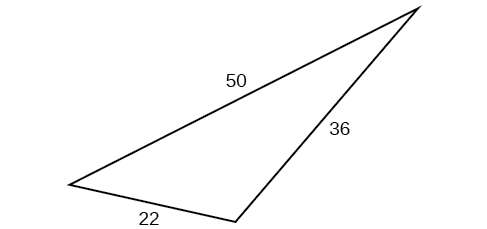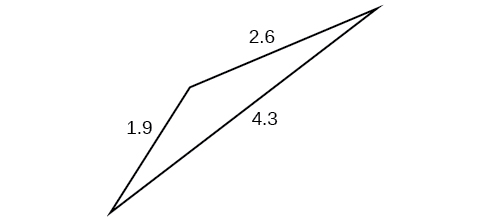1.41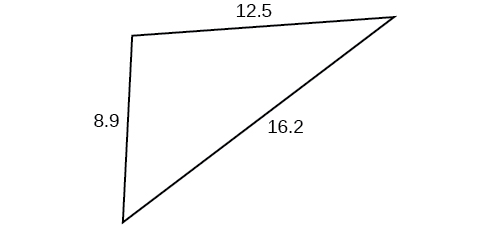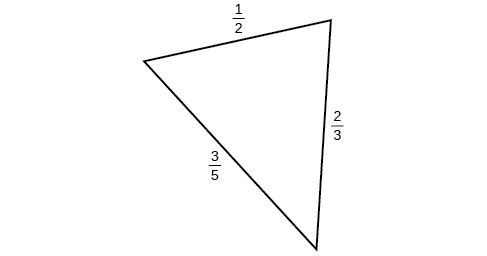0.14

## Extensions

A parallelogram has sides of length 16 units and 10 units. The shorter diagonal is 12 units. Find the measure of the longer diagonal.

The sides of a parallelogram are 11 feet and 17 feet. The longer diagonal is 22 feet. Find the length of the shorter diagonal.

18.3

The sides of a parallelogram are 28 centimeters and 40 centimeters. The measure of the larger angle is 100°. Find the length of the shorter diagonal.

A regular octagon is inscribed in a circle with a radius of 8 inches. (See [link] .) Find the perimeter of the octagon.

48.98

A regular pentagon is inscribed in a circle of radius 12 cm. (See [link] .) Find the perimeter of the pentagon. Round to the nearest tenth of a centimeter.

For the following exercises, suppose that $\text{\hspace{0.17em}}{x}^{2}=25+36-60\mathrm{cos}\left(52\right)\text{\hspace{0.17em}}$ represents the relationship of three sides of a triangle and the cosine of an angle.

Draw the triangle.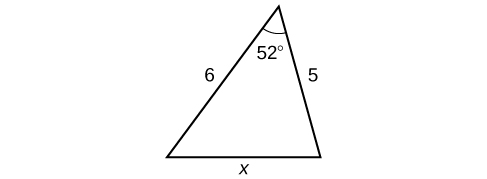Find the length of the third side.

For the following exercises, find the area of the triangle.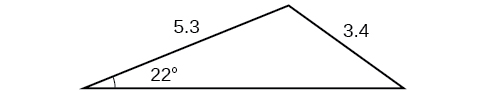7.62

#### Questions & Answers

x exposant 4 + 4 x exposant 3 + 8 exposant 2 + 4 x + 1 = 0
x exposent4+4x exposent3+8x exposent2+4x+1=0
HERVE
How can I solve for a domain and a codomains in a given function?
ranges
EDWIN
Thank you I mean range sir.
Oliver
proof for set theory
don't you know?
Inkoom
find to nearest one decimal place of centimeter the length of an arc of circle of radius length 12.5cm and subtending of centeral angle 1.6rad
factoring polynomial
find general solution of the Tanx=-1/root3,secx=2/root3
find general solution of the following equation
Nani
the value of 2 sin square 60 Cos 60
0.75
Lynne
0.75
Inkoom
when can I use sin, cos tan in a giving question
depending on the question
Nicholas
I am a carpenter and I have to cut and assemble a conventional roof line for a new home. The dimensions are: width 30'6" length 40'6". I want a 6 and 12 pitch. The roof is a full hip construction. Give me the L,W and height of rafters for the hip, hip jacks also the length of common jacks.
John
I want to learn the calculations
where can I get indices
I need matrices
Nasasira
hi
Raihany
Hi
Solomon
need help
Raihany
maybe provide us videos
Nasasira
Raihany
Hello
Cromwell
a
Amie
What do you mean by a
Cromwell
nothing. I accidentally press it
Amie
you guys know any app with matrices?
Khay
Ok
Cromwell
Solve the x? x=18+(24-3)=72
x-39=72 x=111
Suraj
Solve the formula for the indicated variable P=b+4a+2c, for b
Need help with this question please
b=-4ac-2c+P
Denisse
b=p-4a-2c
Suddhen
b= p - 4a - 2c
Snr
p=2(2a+C)+b
Suraj
b=p-2(2a+c)
Tapiwa
P=4a+b+2C
COLEMAN
b=P-4a-2c
COLEMAN
like Deadra, show me the step by step order of operation to alive for b
John
A laser rangefinder is locked on a comet approaching Earth. The distance g(x), in kilometers, of the comet after x days, for x in the interval 0 to 30 days, is given by g(x)=250,000csc(π30x). Graph g(x) on the interval [0, 35]. Evaluate g(5)  and interpret the information. What is the minimum distance between the comet and Earth? When does this occur? To which constant in the equation does this correspond? Find and discuss the meaning of any vertical asymptotes.
The sequence is {1,-1,1-1.....} hasByByByByBy David Martin